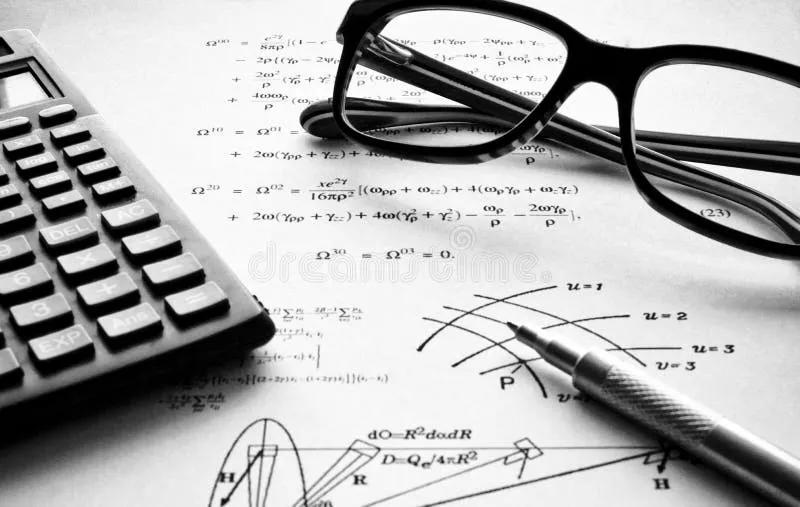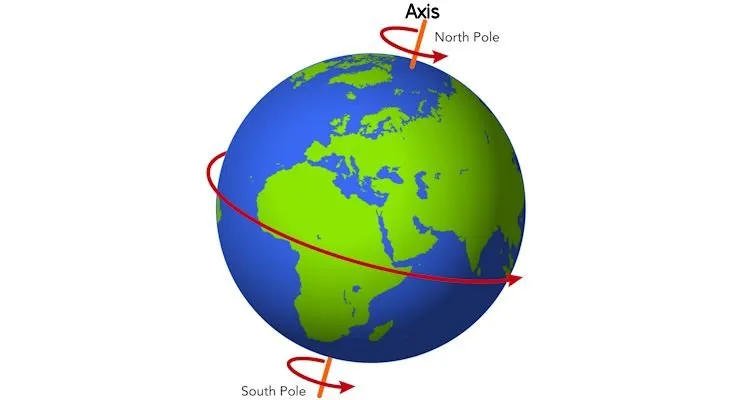# Physics - Classical mechanics - Plane motion exercisesHello it's a me again drifter1! Today we continue with Physics by getting into the promised examples or exercises. We will get into 1 or more examples in each topic of plane motion! So, without further do, let's get started!

### Velocity example:

An object starts at t0=0 in the position (x0, y0) = (2.7m, 3.8m).

It ends up at the position (x, y) = (-4.5m, 8.1m) at t = 4s.

Calculate the vector components, the meter/value and the direction of the average velocity.

The change in time is: Δt = t - t0 = 4 - 0 = 4 sec

At the x-axis we have:

Δx = x - x0 = -4.5 - 2.7 = -7.2 m

and so u avg x = Δx/Δt = -7.2 / 4 = -1.8 m/s

At the y-axis we have:

Δy = y - y0 = 8.1 - 3.8 = 4.3m

and so u avg y = Δy/Δt = 4.3/4 = 1.075 m/s

So, the average velocity meter is:

|u avg| = root[(u avg x)^2 + (u avg y)^2] = root (3.24 + 1.16) = root(4.4) = ~2.1 m/s

and the angle and so direction of the velocity is:

θ = arctan[u avg y/ u avg x] = arctan [1.075/ -1.8] =>

θ = arctan [-0.597] = ~ -0.538 rad or -30.82 degrees.

### Acceleration example:

An object has the following velocity components at t1 = 10s:

ux = 4.5 m/s and uy = 3.2 m/s

In the time period of t1 = 10s to t2 = 20s the object has an average acceleration of 0.55 m/s^2 with an angle of 52 degrees to the x-axis.

At t = 20s find the velocity components, meter and direction.

Because, a avg = 0.55 m/s^2 and the angle is 52 degrees we have the following two equations:

a avg ^2 = a avg x ^2 + a avg y ^2 =>

a avg x^2 + a avg y^2 = 0.3025

and

arctan(a avg y / a avg x) = 52 => tan(52) = a avg y / a avg x =>

-6.053 = a avg y / a avg x

Only the acceleration components are unknown.

By solving this system we end up with:

a avg y = ~ 0.54 m/s^2

a avg x = ~ 0.09 m/s^2

And so the velocity components are (const acceleration motion):

ux = ux0 + a avgx*Δt = 4.5 + 0.09*10 = 5.4 m/s

uy = uy0 + a avgy*Δt = 3.2 + 0.54*10 = 8.6 m/s

This means the the meter is:

|u| = root(ux^2 + uy^2) = root (5.4^2 + 8.6^2) = ~10.15 m/s

and the direction is:

θ = arctan(uy/ux) = arctan(8.6/5.4) = 1.01 rad or 57.86 degrees

### Projectile motion examples:

Example 1

A book slips off a horizontal table with an horizontal velocity (u0x) of 3.6 m/s.

It gets to the ground after 0.5 seconds.Calculate:

1. the height of the table
2. the horizontal distance from the table side to the point it hit the ground
3. the component vectors, meter and direction of the velocity when it hits the ground

Suppose that gravity is equal to g = 10 m/s^2.

1.

The object has no vertical velocity initially (u0y = 0).

The motion in the y-axis is a free falling motion with contant acceleration of g = 10 m/s^2.

This means that can use the equation:

y = y0 + u0y*t - 1/2*g*t^2

where y = 0 cause we hit the ground, u0y = 0, g = 10 m/s^2 and t = 0.5s.

That way we get the height (h = y0):

0 = h + 0 - 1/2*10*0.5^2 =>

h = 1.25 m

2.

The motion in the x-axis is a constant velocity motion and we already know that we hit the ground at t=0.5s. This means that we can use the basic equation for that motion which is:

x = x0 + u0x*t

Supposing the starting position x0 = 0 we end up with:

x = 0 + 3.6*0.5 = 1.8 m

3.

In the x-axis we have:

ux = u0x = 3.6 m/s (constant)

In the y-axis we have:

uy = u0y - g*t (free fall motion)

uy = 0 - 10*0.5 = -5 m/s

which means that we are going down ( yeeeah who didn't know this? :P )

So, the meter of velocity is:

|u| = root (ux^2 + uy^2) = root(37.96) = ~ 6.16 m/s

with an angle of:

φ = arctan(uy/ux) = arctan(-5/3.6) = arctan(-1.38) = ~ - 0.94 rad or -53.85 degrees

which means that we have a slight to heavy angle downwards.

Example 2

A baseball was shot with an angle of 30 degrees and gets catched by the outer player 122m away from the initial point, where the player with the baseball bat was. Suppose that the height where the ball was shot from is the same as the height it was catched at.Calculate:

1. The initial velocity of the ball
2. The maximum height it reached

Suppose that g = 10 m/s^2.

1.

We only know the angle and the distance traveled.

• The motion in the x-axis has a constant velocity
• the motion in the y-axis is a free falling motion

The velocity in each axis can be given by the angle in the beginning and so:

u0x = u0*cos30 = u0*root(3) / 2

u0y = u0*sin30 = u0 / 2

We use the simple distance equation for the x-axis:

x = x0 + u0x*t

where x0 = 0, u0x is unknown, t is unknown and x = 122m

This gives us:

x = 0 + u0x*t = u0*t*root(3)/2 =>

244/root(3) = u0*t  (i)

The distance in the y-axis is:

y = y0 + u0/2*t - 1/2gt^2

We end up at the same height (y = y0) and this gives us:

u0/2 * t = 1/2*10*t^2 =>

u0/2 = 5t =>

u0 = 10t   (ii)

By using (ii) on (i) we get:

244/root(3) = 10*t^2 =>

t^2 = ~ 14.1 => t = ~ 3.75 sec

And so from (ii) the initial velocity is:

u0 = 10*3.75 = 37.5 m/s

For each axis we have:

uox = uo*root(3) / 2 = ~32.43 m/s

u0y = u0/2 = 18.75 m/s

The angle is known and is 30 degrees.

2.

To calculate the height we will use the simple equation from before:

y = y0 + u0y*t - 1/2*g*t^2

Now the starting point is 0, but the end point (height) is not zero and so:

h = 18.75*t - 1/2*10*t^2.

Now we have to find the time.

From the velocity equation (with uy = 0) we have:

uy = u0y - g*t => 0 = 18.75 - 10*t => t = 1.875 s

That way h = ~17.58 m

### Smooth Circular Motion Examples:

Example 1

A theme park wheel has a radius of R = 14 m.

The linear velocity of an passenger (and so the outside) is 9 m/s.Calculate:

1. The meter and direction of the acceleration when the passenger is at the bottom
2.  -||- at the top

Suppose that gravity is g = 9.8 m/s^2.

1.

The acceleration of gravity pushes the passenger down and the (angular or centrifuge) acceleration of the wheel's rotation pushes him up.

The period of rotation is:

u = 2πR / T => T = 2πR/u => T = 2π*14/9 => T = 9.77 sec

The acceleration from the wheel is:

a rad= 4π^2R / T^2 = 4π^2*14/9.77^2 = ~5.79  m/s^2

And so the total acceleration is:

a = a rad - g = 5.79 - 9.8 = -0.401 m/s^2

which means that the passenger gets pushed down!

2.

At the top the acceleration from the wheel is the same and so because gravity changes we now have a total acceleration of a = 5.79 + 9.8 = 15.59 m/s^2 downwards.

Example 2

The earth has an radius of 6.38 x 10^6 m and needs 24h to do a rotation of the axis.

The earth has an radius of 1.49 x 10^11 (actually not circular but anyway) and needs 365 days to do a rotation (orbit) of the sun.Calculate the angular acceleration of an object at the equator of the earth and the angular acceleration of the earth's motion and compare the results.

24 hours are 86400 seconds.

365 days are 3.154 x 10^7 seconds.

The angular acceleration of the earth's axis rotation is:

a rad = ω^2*R = 4π^2R / T^2 = 4π^2*6.38 x 10^6 / 86400^2 =>

The angular acceleration of the earth's rotation to the sun is:

a rad = 4π^2R / T^2 = 4π^2*1.49 x 10^11 / (3.154 x 10^7) =>

Which means that we are actually travelling much faster around the sun (together with the earth) then we travel around the earth's axis!

### Image Sources:https://www.polyvore.com/cgi/img-thing?.out=jpg&size=l&tid=6530316And this is actually it for today and I hope that you enjoyed it!
From next time in Physics, we wil get into Forces and Newton's Laws!

Bye!

H2
H3
H4
3 columns
2 columns
1 column